# Please Explain the Answer Question 2 (Parts A-C, 20 points): Use the energy diagrams shown below...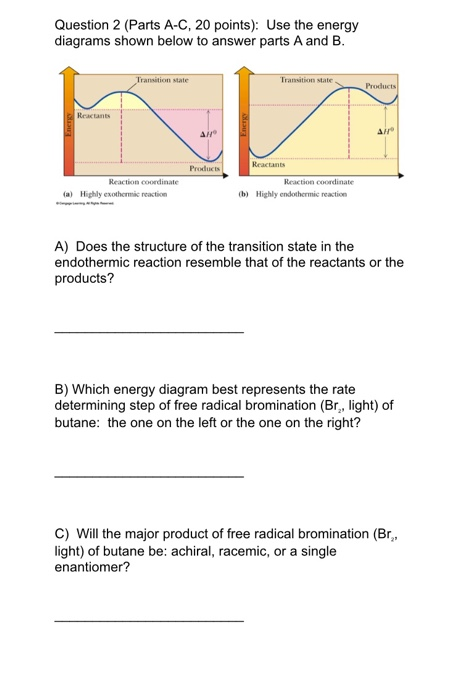Question 2 (Parts A-C, 20 points): Use the energy diagrams shown below to answer parts A and B. on Products Rectants Energy Products Reactants Reaction coordinate (a) Highly exothermic reaction Reaction coordinate (b) Highly endothermic reaction A) Does the structure of the transition state in the endothermic reaction resemble that of the reactants or the products? B) Which energy diagram best represents the rate determining step of free radical bromination (Br,, light) of butane: the one on the left or the one on the right? C) Will the major product of free radical bromination (Br. light) of butane be: achiral, racemic, or a single enantiomer?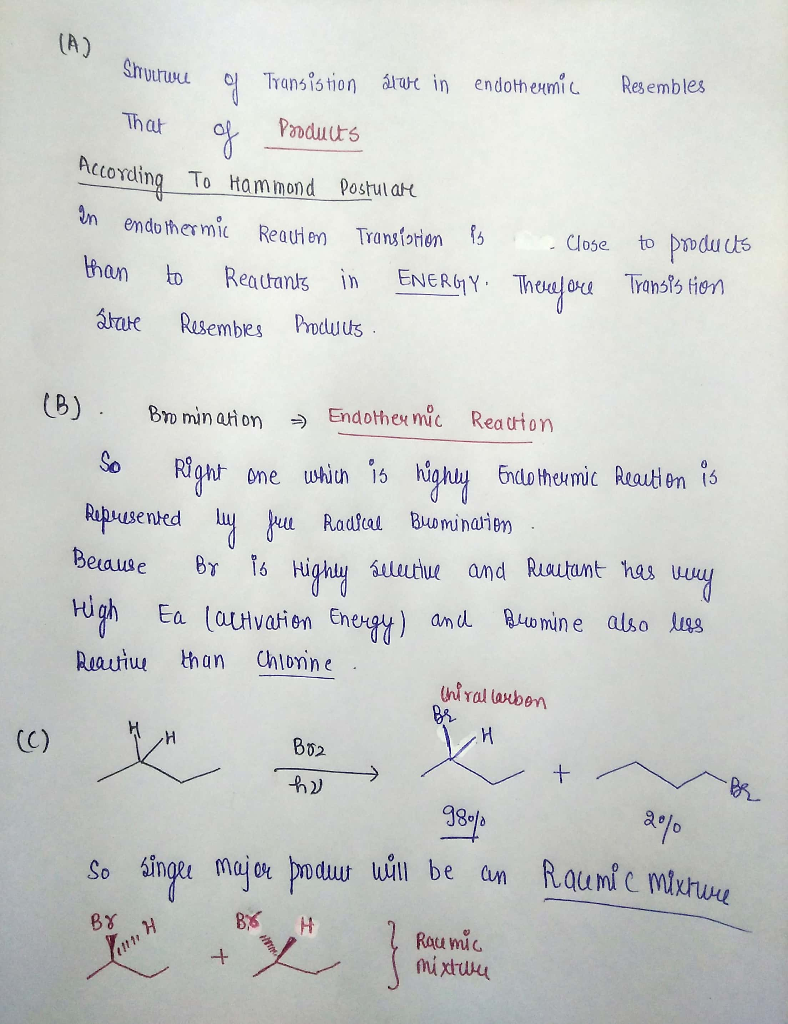#### Earn Coin

Coins can be redeemed for fabulous gifts.

Similar Homework Help Questions
• ### 125% Zoom T Insert HE Table T Text View Chart Shape Media Comment Collaborate Format Document...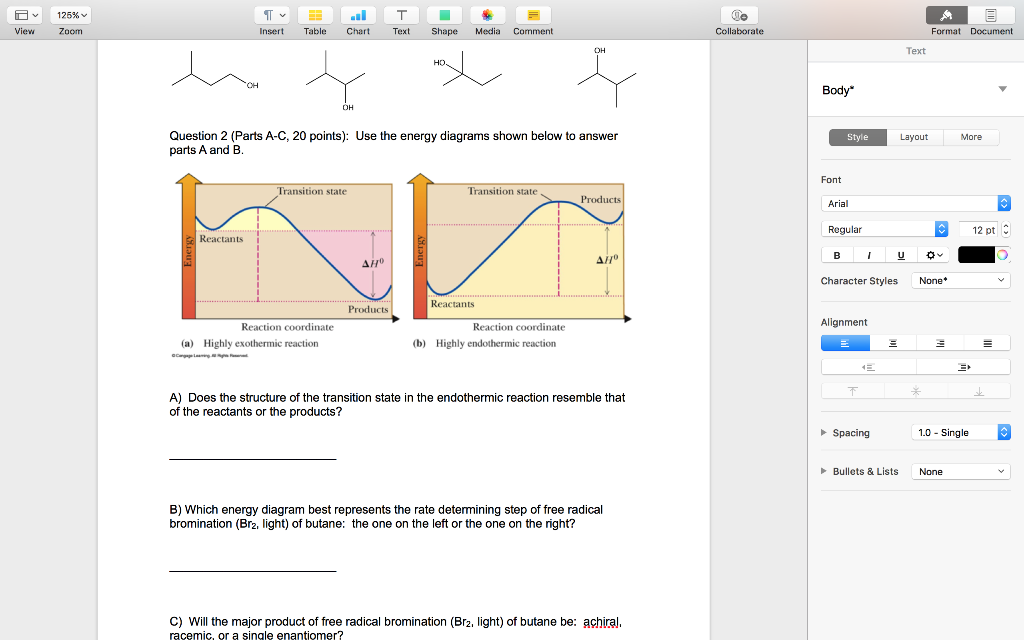125% Zoom T Insert HE Table T Text View Chart Shape Media Comment Collaborate Format Document Text Body Question 2 (Parts A-C, 20 points): Use the energy diagrams shown below to answer parts A and B. Style Layout More Font Transition state Transition state - Products Arial ..... ............ Regular 12 pt Reactants Energy Energy Character Styles None* Reactants Products Alignment Reaction coordinate (a) Highly exothermic reaction Reaction coordinate (b) Highly endothermic reaction T oke A) Does the structure of...

• ### 3. Please answer the following questions concerning the two energy diagrams depicted below. (10 pts) Energy...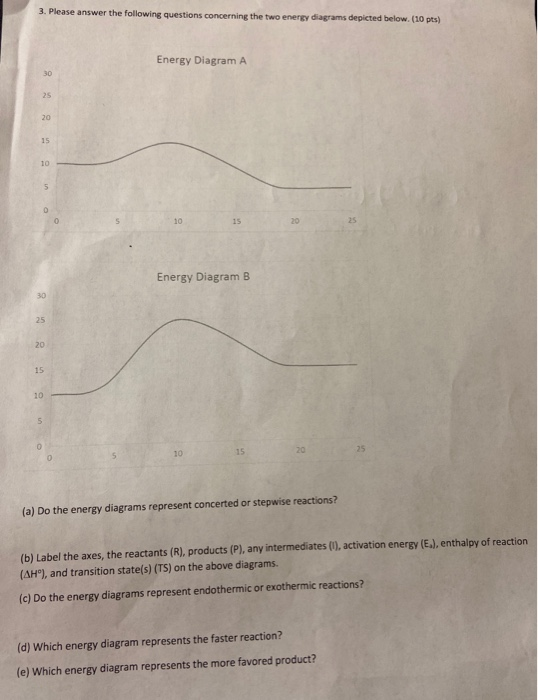3. Please answer the following questions concerning the two energy diagrams depicted below. (10 pts) Energy Diagram A 20 Energy Diagram B (a) Do the energy diagrams represent concerted or stepwise reactions? (b) Label the axes, the reactants (R), products (P), any intermediates (1), activation energy (E.), enthalpy of reaction (AH"), and transition state(s) (TS) on the above diagrams. (c) Do the energy diagrams represent endothermic or exothermic reactions? (d) Which energy diagram represents the faster reaction? (e) Which energy...

• ### 3. Please answer the following questions concerning the two energy diagrams depicted below. (10 pts) Energy...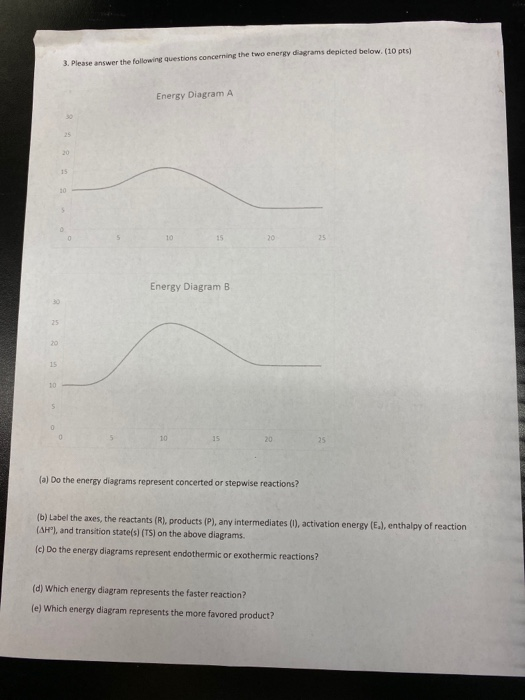3. Please answer the following questions concerning the two energy diagrams depicted below. (10 pts) Energy Diagram A Energy Diagram B (a) Do the energy diagrams represent concerted or stepwise reactions? (b) Label the axes, the reactants (R), products (P), any intermediates (1), activation energy (E.), enthalpy of reaction (AH'), and transition state(s) (TS) on the above diagrams. (C) Do the energy diagrams represent endothermic or exothermic reactions? (d) Which energy diagram represents the faster reaction? (e) Which energy diagram...

• ### Name: 17. Consider the two step reaction A B-C (shown below). Which stepp is the rate...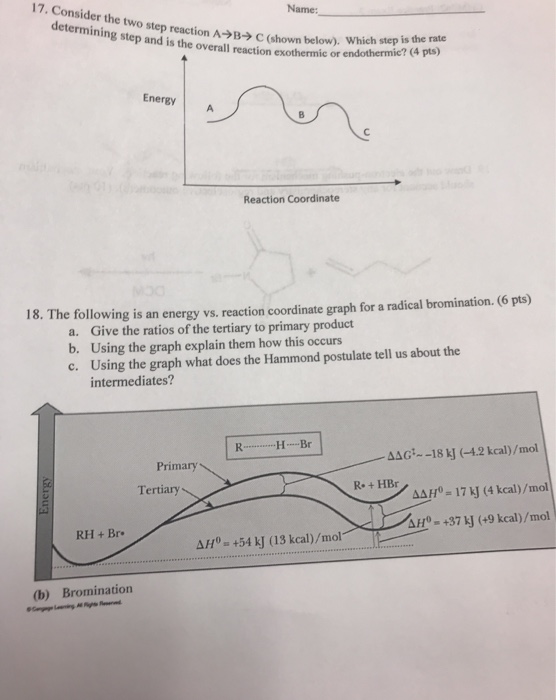Name: 17. Consider the two step reaction A B-C (shown below). Which stepp is the rate determining step and is the overall reaction exothermic or endothermic? (4 pts) Energy A Reaction Coordinate 18. The following is an energy vs. reaction coordinate graph for a radical bromination. (6 pts) a. Give the ratios of the tertiary to primary product b. Using the graph explain them how this occurs c. Using the graph what does the Hammond postulate tell us about the...

• ### Model 1 - Potential Energy Diagrams 1) The energy (enthalpy) change of a reaction can be...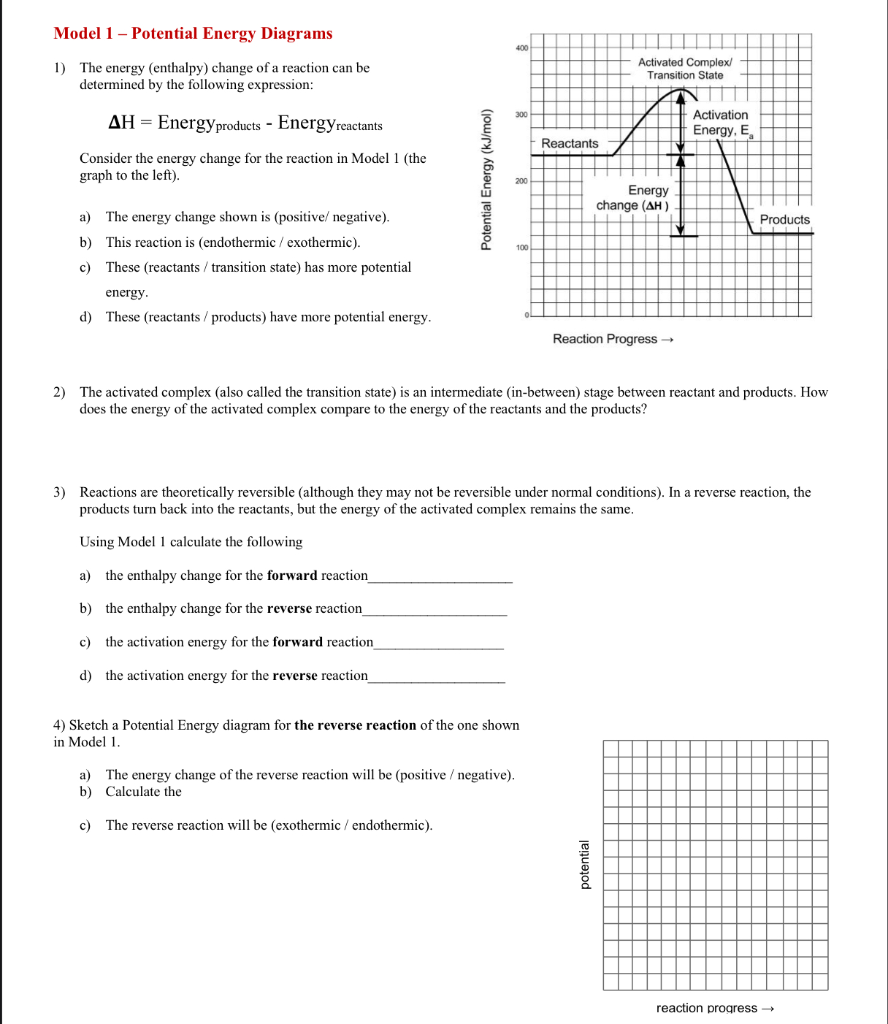Model 1 - Potential Energy Diagrams 1) The energy (enthalpy) change of a reaction can be determined by the following expression: Activated Complex Transition State AH = Energy products - Energy reactants Activation Energy, E Reactants Consider the energy change for the reaction in Model 1 (the graph to the left). Potential Energy (kJ/mol) Energy change (AH) Products a) The energy change shown is (positive/negative). b) This reaction is (endothermic / exothermic). c) These (reactants / transition state) has more...

• ### 3. Please answer the following questions concerning the two energy diagrams depicted below. (10 pts) Energy...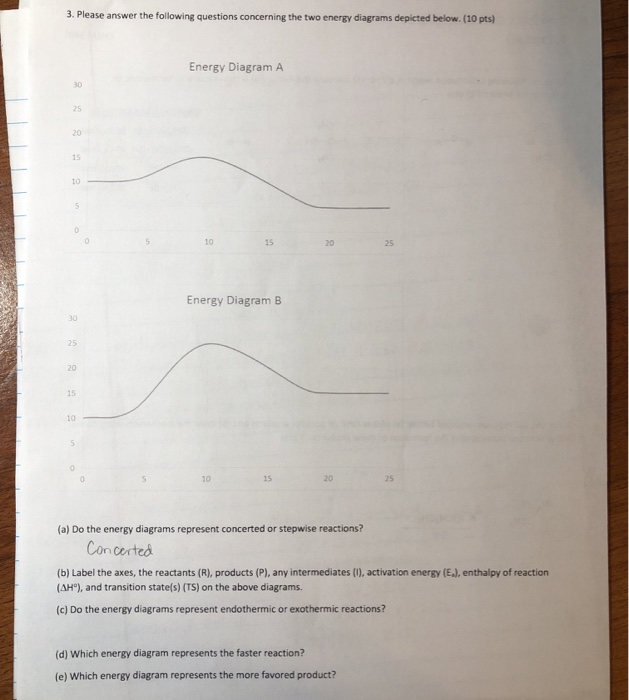3. Please answer the following questions concerning the two energy diagrams depicted below. (10 pts) Energy Diagram A 30 25 20 15 10 0 10 15 20 25 Energy Diagram B 30 25 20 15 10 15 20 25 10 (a) Do the energy diagrams represent concerted or stepwise reactions? Concerted (b) Label the axes, the reactants (R), products (P), any intermediates (), activation energy (E), enthalpy of reaction (AH), and transition state(s) (TS) on the above diagrams. (c) Do...

• ### Can somebody explain and answer 23 and 24 9:55 PM Sat Nov 2 .37% < 0...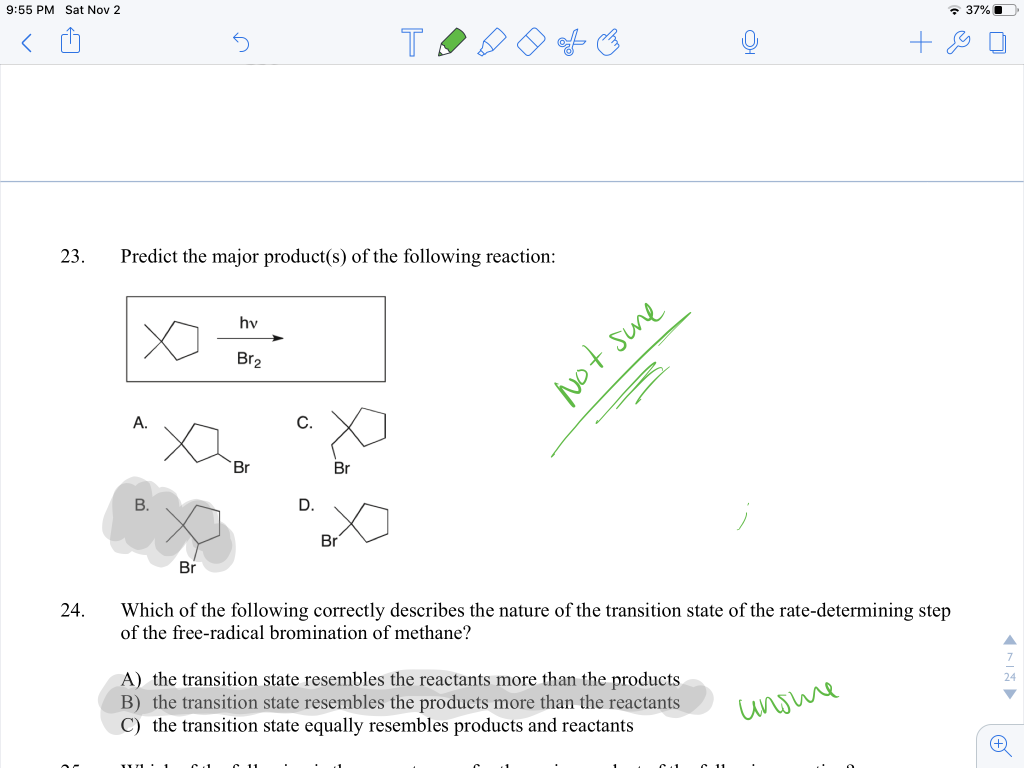Can somebody explain and answer 23 and 24 9:55 PM Sat Nov 2 .37% < 0 5 TODO of ☺ ☺ + \$ 23. Predict the major product(s) of the following reaction: sure Not Br 24. Which of the following correctly describes the nature of the transition state of the rate-determining step of the free-radical bromination of methane? A) the transition state resembles the reactants more than the products B) the transition state resembles the products more than the reactants...

• ### ​​​​​​​Please answer all two questions and please explain! I'm completely lost at the moment and I'm...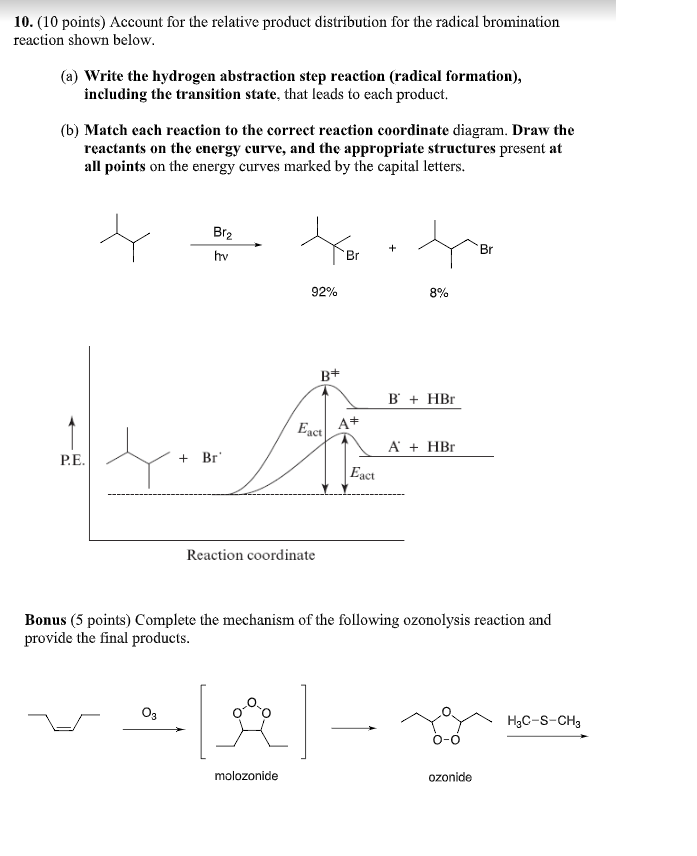​​​​​​​Please answer all two questions and please explain! I'm completely lost at the moment and I'm not sure how to solve it. Please do it step by step if possible. 10. (10 points) Account for the relative product distribution for the radical bromination reaction shown below. (a) Write the hydrogen abstraction step reaction (radical formation), including the transition state, that leads to each product. (b) Match each reaction to the correct reaction coordinate diagram. Draw the reactants on the energy...

• ### Please answer all 3 questions QUESTION 23 Which of the following statements are true? 1. ethanol...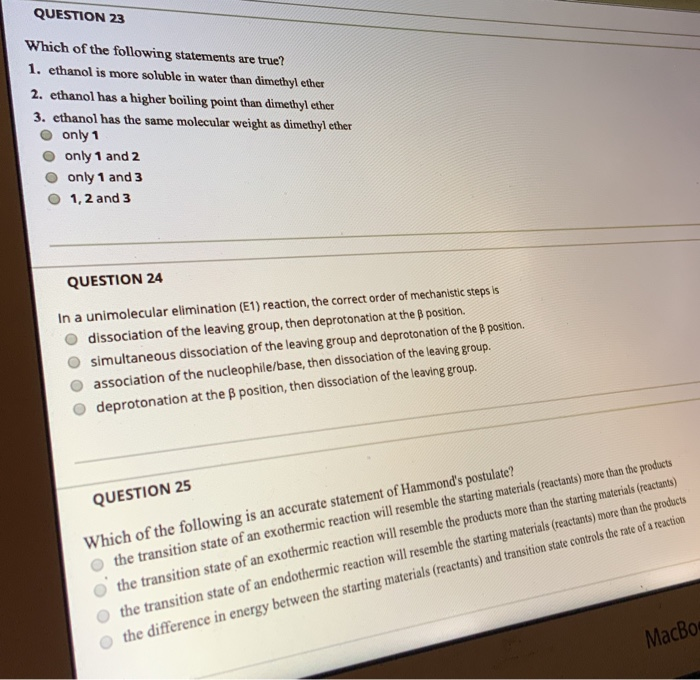Please answer all 3 questions QUESTION 23 Which of the following statements are true? 1. ethanol is more soluble in water than dimethyl ether 2. ethanol has a higher boiling point than dimethyl ether 3. ethanol has the same molecular weight as dimethyl ether only 1 only 1 and 2 only 1 and 3 1, 2 and 3 QUESTION 24 In a unimolecular elimination (E1) reaction, the correct order of mechanistic steps is dissociation of the leaving group, then deprotonation...

• ### Justify Answers. Consider the energy diagram below showing the conversion of A to G a) How...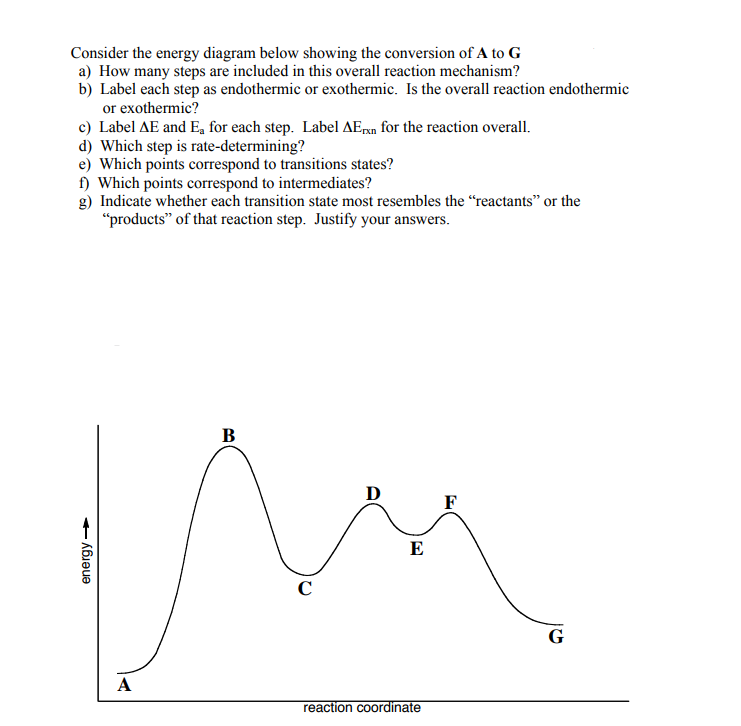Justify Answers. Consider the energy diagram below showing the conversion of A to G a) How many steps are included in this overall reaction mechanism? b) Label each step as endothermic or exothermic. Is the overall reaction endothermid or exothermic? c) Label ΔE and Ea for each step. Label ΔEnn for the reaction overall. d) Which step is rate-determining? e) Which points correspond to transitions states? f) Which points correspond to intermediates? g) Indicate whether each transition state most resembles...

Free Homework App